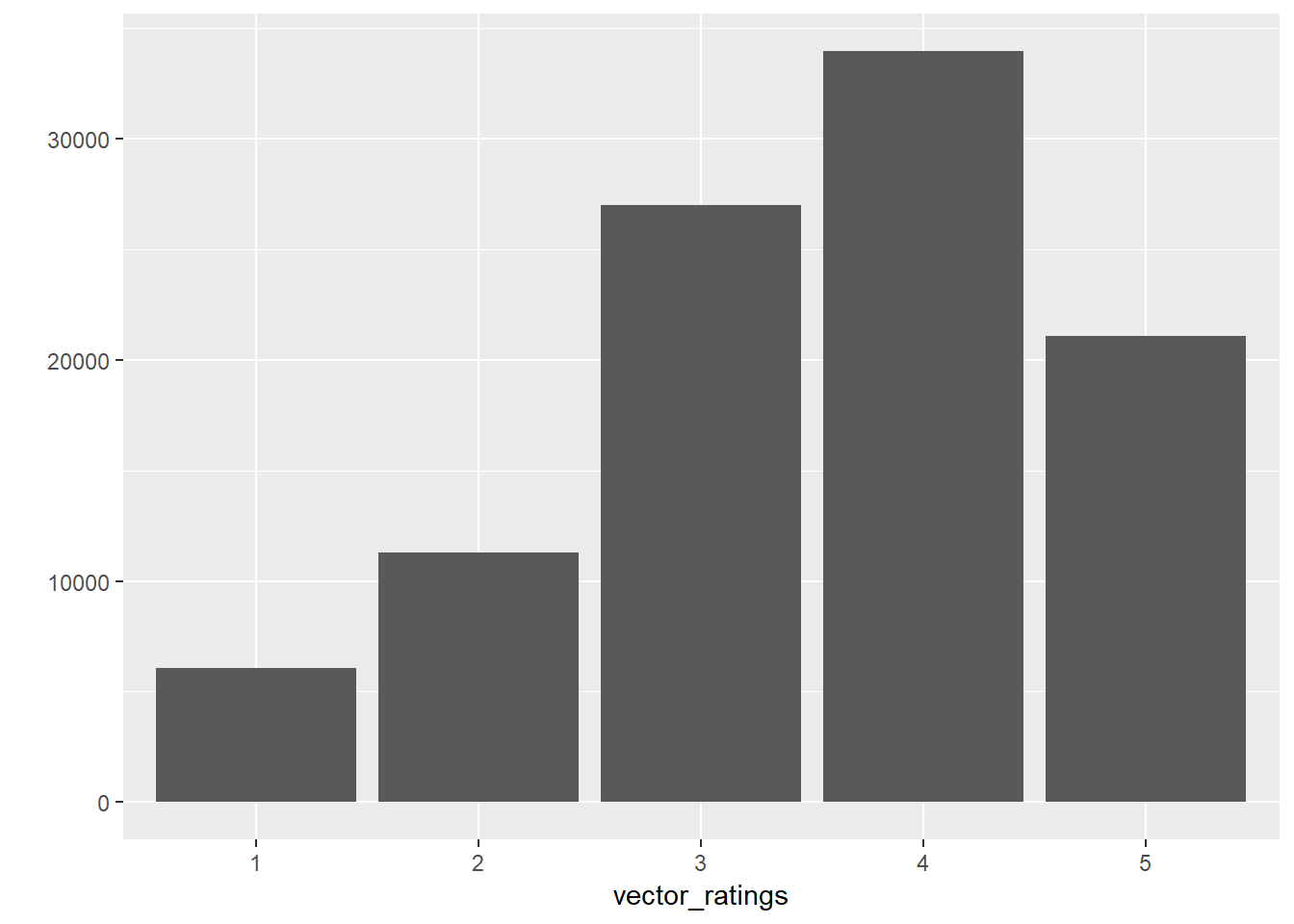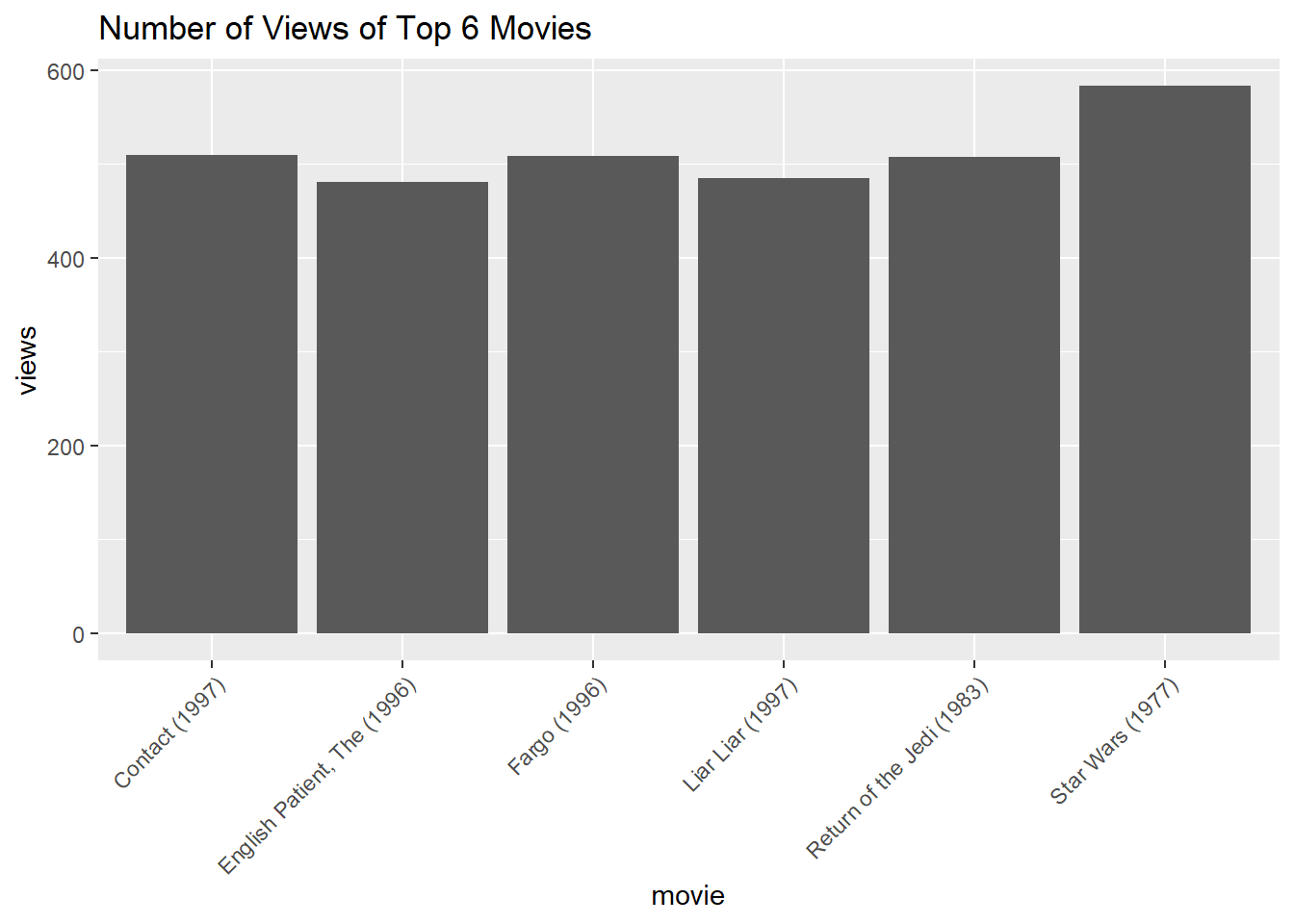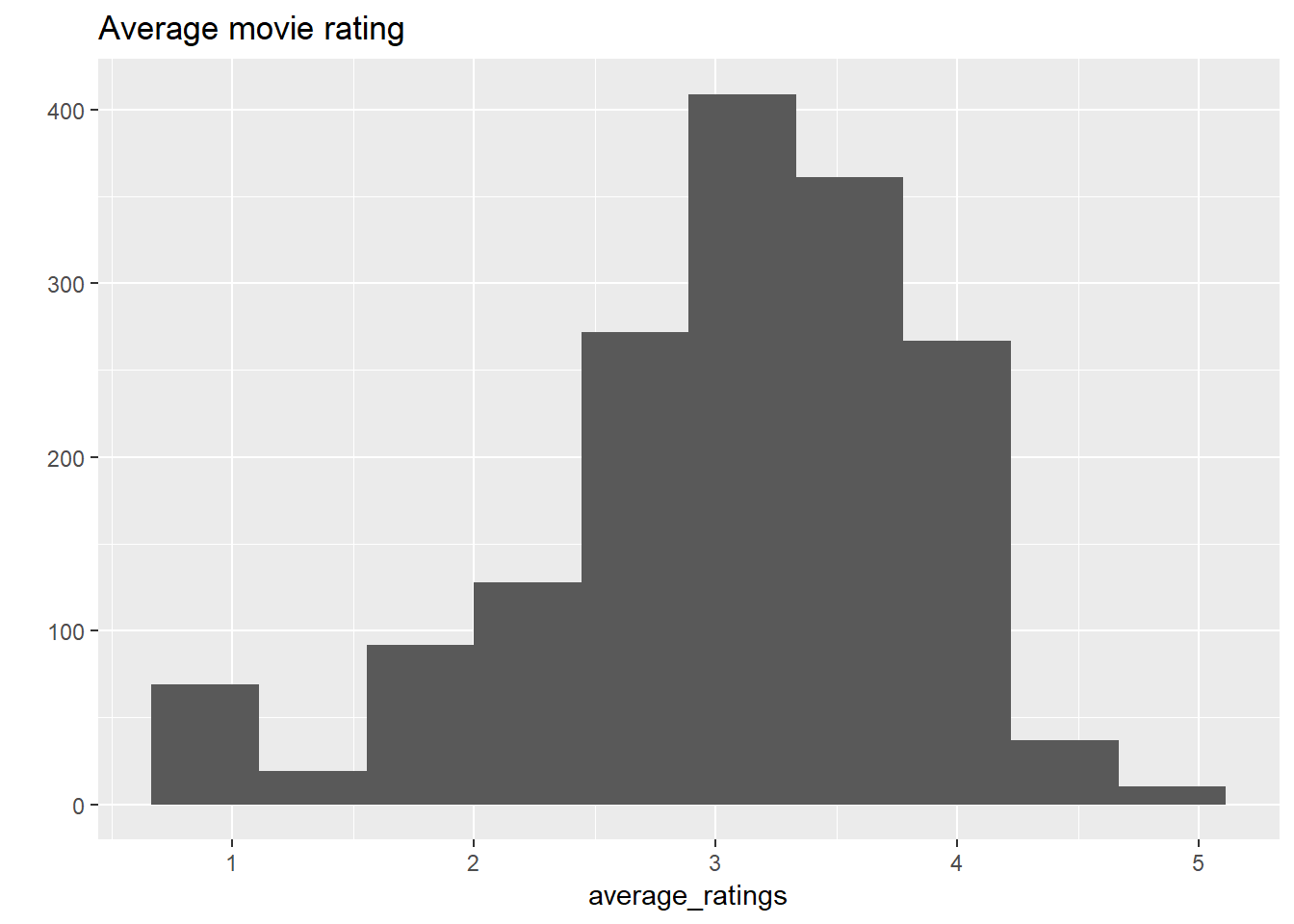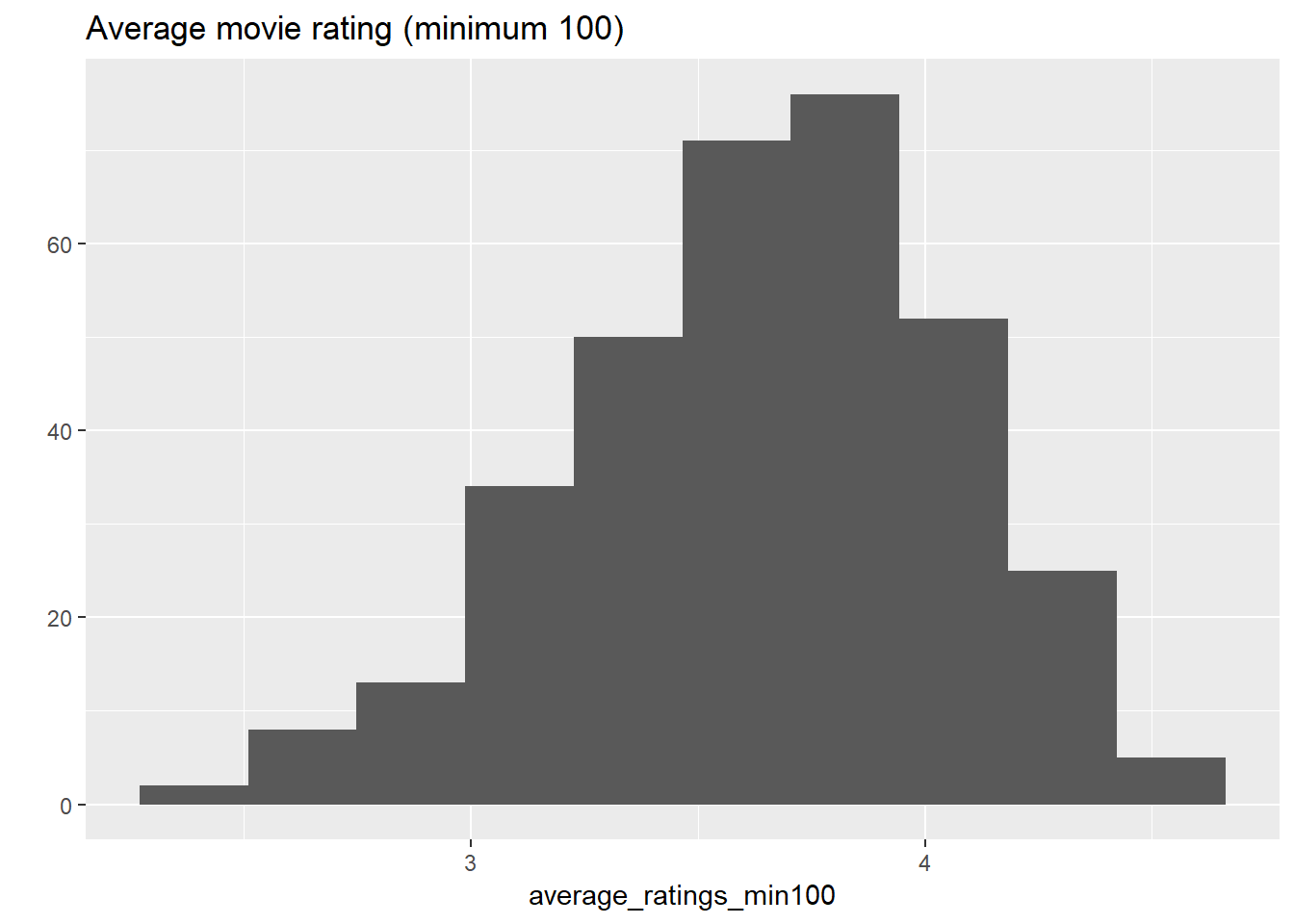# Overview:

In the following R code, IBCF system is applied to MovieLense data

### import libraries

library(recommenderlab)
## Loading required package: Matrix
## Loading required package: arules
##
## Attaching package: 'arules'
## The following objects are masked from 'package:base':
##
##     abbreviate, write
## Loading required package: proxy
##
## Attaching package: 'proxy'
## The following object is masked from 'package:Matrix':
##
##     as.matrix
## The following objects are masked from 'package:stats':
##
##     as.dist, dist
## The following object is masked from 'package:base':
##
##     as.matrix
## Loading required package: registry
library(ggplot2)
library(recosystem)
set.seed(1)
library(devtools)
install_github(repo = "tarashnot/SlopeOne", username = "tarashnot")
## Skipping install of 'SlopeOne' from a github remote, the SHA1 (fa91818f) has not changed since last install.
##   Use force = TRUE to force installation
install_github(repo = "tarashnot/SVDApproximation", username = "tarashnot")
## Skipping install of 'SVDApproximation' from a github remote, the SHA1 (b53f26e5) has not changed since last install.
##   Use force = TRUE to force installation

### import the MovieLense data

data(MovieLense)
MovieLense
## 943 x 1664 rating matrix of class 'realRatingMatrix' with 99392 ratings.

### View the size of the MovieLense data

object.size(MovieLense)
## 1409432 bytes
object.size(as(MovieLense, "matrix"))
## 12761360 bytes

### converting the matrix into vector to see values

vector_ratings <- as.vector(MovieLense@data)
unique(vector_ratings)
##  5 4 0 3 1 2
table(vector_ratings)
## vector_ratings
##       0       1       2       3       4       5
## 1469760    6059   11307   27002   33947   21077

### removing the null values and turning vector into factors

vector_ratings <- vector_ratings[vector_ratings != 0]
vector_ratings <- factor(vector_ratings)

qplot(vector_ratings)### calculating and visualizing which movies have been viewed

views_per_movie <- colCounts(MovieLense)

table_views <- data.frame(
movie = names(views_per_movie),
views = views_per_movie
)

table_views <- table_views[order(table_views\$views,
decreasing = TRUE), ]

ggplot(table_views[1:6, ], aes(x=movie, y=views)) +
geom_bar(stat = "identity") +
theme(axis.text.x = element_text(angle = 45, hjust = 1)) +
ggtitle("Number of Views of Top 6 Movies")### visualizing the average movie score

average_ratings <- colMeans(MovieLense)

qplot(average_ratings) +
stat_bin(bins = 10) +
ggtitle("Average movie rating")
## stat_bin() using bins = 30. Pick better value with binwidth.### view the average ratings of only movies with 100 views minimum

average_ratings_min100 <- average_ratings[views_per_movie >= 100]

qplot(average_ratings_min100) +
stat_bin(bins = 10) +
ggtitle("Average movie rating (minimum 100)")
## stat_bin() using bins = 30. Pick better value with binwidth.### selecting only data with enough ratings and power users

# greater than 100 views
# only accounting for users that have rated at least 50 movies
ratings_movies <- MovieLense[rowCounts(MovieLense) > 50,
colCounts(MovieLense) > 100]
ratings_movies
## 560 x 332 rating matrix of class 'realRatingMatrix' with 55298 ratings.
#average ratings per user
avg_ratings_user <- rowMeans(ratings_movies)

### normalize the user ratings to zero

ratings_movies_normalize <- normalize(ratings_movies)

### splitting the data into training and testing sets

which_train <- sample(x = c(TRUE, FALSE), size = nrow(ratings_movies),
replace = TRUE, prob = c(0.8, 0.2))

train <- ratings_movies[which_train, ]
test <- ratings_movies[!which_train, ]

### use k-fold to split the users into 5 groups

which_set <- sample(x = 1:5, size=nrow(ratings_movies),
replace = TRUE)
for(i in 1:5) {
which_train <- which_set == i
train <- ratings_movies[which_train, ]
test <- ratings_movies[!which_train, ]
}

### establishing the Item Based Collaborative Filtering recommender model

model <- Recommender(data = train, method = "IBCF",
parameter = list(k=30))
model
## Recommender of type 'IBCF' for 'realRatingMatrix'
## learned using 111 users.

### apply model onto the test set (IBCF model)

# number of items to recommend
n_recommend <- 5

predicted <- predict(object = model, newdata = test, n = n_recommend)
predicted
## Recommendations as 'topNList' with n = 5 for 449 users.

### now, recommend movies for each user in the test set (IBCF model)

recommender_matrix <- sapply(predicted@items, function(x){
colnames(ratings_movies)[x]
})

recommender_matrix[, 2:4]
##      2
## [1,] "Babe (1995)"
## [2,] "Natural Born Killers (1994)"
## [3,] "Mystery Science Theater 3000: The Movie (1996)"
## [4,] "Frighteners, The (1996)"
## [5,] "Event Horizon (1997)"
##      3                                5
## [1,] "Craft, The (1996)"              "Ace Ventura: Pet Detective (1994)"
## [2,] "Aladdin (1992)"                 "Cape Fear (1991)"
## [3,] "While You Were Sleeping (1995)" "Dumbo (1941)"
## [4,] "Emma (1996)"                    "Little Women (1994)"
## [5,] "Michael (1996)"                 "Peacemaker, The (1997)"

# Summary

To summarize, it was amazing how much more data the distributed recommender system could handle. 20 Million rows with ease! In the future, if I had a dataset of more than 1 million rows I would consider databricks rather than a local recommender system.